# Draak Update

Below is gameplay video of a prototype of the puzzle game “Draak” that I am working on. Draak will be an iPad-only iOS game; the prototype below is written to Windows in C# on top of MonoGame.

The initial idea for this game was about the art. I thought it would be cool to make a game that looks like an animated version of one of M. C. Escher’s tessellations, particularly one that involves a similarity symmetry. So I lifted mechanics from a 1990s era game that I always felt was a good game trapped in the typical square lattice for which it was particularly ill-suited. The old game’s Euclidean lay-out wasted a lot of space.

A lot of the work in the above may not be immediately apparent (which is good I think). Specifically, there are actually two shapes of butterflies in the above not just one. They look like this: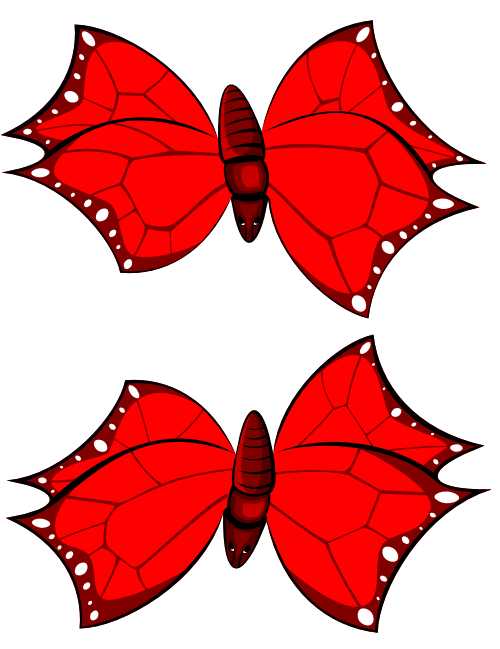and are arrayed as in a checkerboard with a 90 degree rotation applied to cells of opposite parity — the geometry might be clearer here in which I create these butterfly tiles unadorned with my tool EscherDraw (before I had beziers working in EscherDraw) Thus any time a butterfly moves to a cell with opposite parity I need to not only handle the scale and rotation tweening imposed by the spiral lattice, I also have to simulatenously do the 90 degree rotation imposed by the butterfly tiles and simultaneously morph the actual sprites above from one to the other.

I created the above sprites as vector art using tile outlines exported from Escher draw as SVG and wrote a Python script that morphs SVG as the publically available software for morphing vector graphics is surprisingly non-existent. I am going to post about my vector morphing code here soon, I just need to clean it up for use by people other than me.

# Beziers in EscherDraw Video

Creating the draak logo in EscherDraw with bezier curves:

# My Code for Doing Two Things That Sooner or Later You Will Want to do with Bezier Curves

I just added full cubic bezier curve support to the vector tessellation creation tool I am developing (see here). I’ll include some video at a later date (Update: here) showing this feature in action but first I want to document and make publicly available some of the code involved: namely, a bezier class in C# that supports two functions that are not the easiest things in the world to implement. Both of these functions come up as soon as you try to do anything non-trivial with beziers and the literature online can be unhelpful.

My code is here: link.

The first function is splitting a cubic bezier into two curves at a given t parameter such that the concatenation of the two curves is congruent to the original. This turns out to be easy; however, I could find no actual code on offer, rather just descriptions of code. There is good coverage elsewhere on the theory so I won’t go into it deeply here, but, briefly, splitting a bezier is easy because how one does so follows naturally from the sort of recursive definition of the bezier that is implied by De Casteljau’s algorithm. Anyway here is my code:

```...
public Tuple<Bezier,Bezier> Split(double t) {
PointD E = Interpolate(t, CtrlPoint1, CtrlPoint2);
PointD F = Interpolate(t, CtrlPoint2, CtrlPoint3);
PointD G = Interpolate(t, CtrlPoint3, CtrlPoint4);
PointD H = Interpolate(t, E, F);
PointD J = Interpolate(t, F, G);
PointD K = Interpolate(t, H, J);

return new Tuple<Bezier, Bezier>(
new Bezier(CtrlPoint1, E, H, K),
new Bezier(K, J, G, CtrlPoint4)
);
}

static private PointD Interpolate(double t, PointD pt1, PointD pt2) {
double x = (1.0 - t) * pt1.X + t * pt2.X;
double y = (1.0 - t) * pt1.Y + t * pt2.Y;
return new PointD(x, y);
}
....
```

The other function is considerably harder: finding the nearest point on a bezier curve to a given point. If you scan the literature (i.e. perform the google search) you will find that what most people end up using is some code from an article in the book Graphics Gems I, “Solving the Nearest-Point-On-Curve Problem” by Philip J. Schneider. I, however, have a couple of problems with this code (1) I don’t really understand it, (2) it is long and I need to port it to C#, and (3) someone on the internet is claiming that it is incorrect but given (1) I can’t really evaluate the claim; see the comment that begins “There seem to be errors in the ControlPolygonFlatEnough function […]” in the C code here.

But more relevantly I just don’t get this code on a fundamental level. The nearest point on a bezier problem is difficult to solve mathematically but it is easy to formulate mathematically. I think that the Graphics Gems code obscures the straightforward aspect of how this problem can be formulated. Further, I don’t have a problem using code that I don’t understand; however, if I am going to port an opaque block of code from one language to another I’d like to keep that portion of code to a minimum and I can do this easily by structuring my code around the formulation of the problem that I understand.

The formulation I am talking about is as follows, if B(t) is a particular cubic bezier function, B’(t) is its derivative, and P is an arbitrary point then [B(t) – P] ⋅ B’(t) = 0 when t is the parameter of the closest point on B to P. [B(t) – P] is a vector that points from P towards some point on the curve and B’(t) is a vector that is tangent to the curve at this point, if the distance is going to be a minimum then these two vectors will be perpendicular and thus their dot product will be zero. Since B(t) is cubic, its derivative will be quadratic and thus when we take the scalar product of [B(t) – P] and B’(t) we will end up with a quintic equation of one variable. This leads naturally to a nearest point function that looks like the following on the top level:

``` public Tuple<PointD, double> FindNearestPoint(PointD pt)
{
var polyQuintic = GetNearestBezierPtQuintic(
_points.X, _points.Y,
_points.X, _points.Y,
_points.X, _points.Y,
_points.X, _points.Y,
pt.X, pt.Y
);
List<Complex> roots = FindAllRoots(polyQuintic);

// Filter out roots with nonzero imaginary parts and roots
// with real parts that are not between 0 and 1.
List<double> candidates = roots.FindAll(
root => root.Real > 0 && root.Real <= 1.0 && Math.Abs(root.Imaginary) < ROOT_EPS
).Select(
root => root.Real
).ToList();

// add t=0 and t=1 ... the edge cases.
candidates.Add(0.0);
candidates.Add(1.0);

// find the candidate that yields the closest point on the bezier to the given point.
double t = double.NaN;
PointD output = new PointD(double.NaN,double.NaN);
double minDistance = double.MaxValue;
foreach (double candidate in candidates)
{
var ptAtCandidate = GetPoint(candidate);
double distance = DistSqu(ptAtCandidate, pt);
if (distance < minDistance)
{
minDistance = distance;
t = candidate;
output = ptAtCandidate;
}
}

return new Tuple<PointD, double>(output, t);
}
```

which reduces the problem to implementing GetNearestBezierPtQuintic() and a numeric root finding function for polynomials, given that quintic equations cannot be solved via a closed-form formula like the quadratic equation.

GetNearestBezierPtQuintic() –which returns the coefficients of [B(t) – P] ⋅ B’(t) when fully expanded given P and the control points of — turns out to be a little two much algebra to work out comfortably by hand, so I ran the open source symbolic mathematics application Sage on this Sage script yielding the following when translated into C#: (For the first time on the internet! … as far as I can tell)

```static private List<Complex> GetNearestBezierPtQuintic(double x_0, double y_0, double x_1, double y_1,
double x_2, double y_2, double x_3, double y_3, double x, double y)
{
double t5 = 3 * x_0 * x_0 - 18 * x_0 * x_1 + 27 * x_1 * x_1 + 18 * x_0 * x_2 - 54 * x_1 * x_2 + 27 * x_2 * x_2 -
6 * x_0 * x_3 + 18 * x_1 * x_3 - 18 * x_2 * x_3 + 3 * x_3 * x_3 + 3 * y_0 * y_0 - 18 * y_0 * y_1 +
27 * y_1 * y_1 + 18 * y_0 * y_2 - 54 * y_1 * y_2 + 27 * y_2 * y_2 - 6 * y_0 * y_3 + 18 * y_1 * y_3 -
18 * y_2 * y_3 + 3 * y_3 * y_3;
double t4 = -15 * x_0 * x_0 + 75 * x_0 * x_1 - 90 * x_1 * x_1 - 60 * x_0 * x_2 + 135 * x_1 * x_2 -
45 * x_2 * x_2 + 15 * x_0 * x_3 - 30 * x_1 * x_3 + 15 * x_2 * x_3 - 15 * y_0 * y_0 + 75 * y_0 * y_1 -
90 * y_1 * y_1 - 60 * y_0 * y_2 + 135 * y_1 * y_2 - 45 * y_2 * y_2 + 15 * y_0 * y_3 - 30 * y_1 * y_3 +
15 * y_2 * y_3;
double t3 = 30 * x_0 * x_0 - 120 * x_0 * x_1 + 108 * x_1 * x_1 + 72 * x_0 * x_2 - 108 * x_1 * x_2 +
18 * x_2 * x_2 - 12 * x_0 * x_3 + 12 * x_1 * x_3 + 30 * y_0 * y_0 - 120 * y_0 * y_1 +
108 * y_1 * y_1 + 72 * y_0 * y_2 - 108 * y_1 * y_2 + 18 * y_2 * y_2 - 12 * y_0 * y_3 + 12 * y_1 * y_3;
double t2 = 3 * x * x_0 - 30 * x_0 * x_0 - 9 * x * x_1 + 90 * x_0 * x_1 - 54 * x_1 * x_1 + 9 * x * x_2 -
36 * x_0 * x_2 + 27 * x_1 * x_2 - 3 * x * x_3 + 3 * x_0 * x_3 + 3 * y * y_0 - 30 * y_0 * y_0 - 9 * y * y_1 +
90 * y_0 * y_1 - 54 * y_1 * y_1 + 9 * y * y_2 - 36 * y_0 * y_2 + 27 * y_1 * y_2 - 3 * y * y_3 + 3 * y_0 * y_3;
double t1 = -6 * x * x_0 + 15 * x_0 * x_0 + 12 * x * x_1 - 30 * x_0 * x_1 + 9 * x_1 * x_1 - 6 * x * x_2 +
6 * x_0 * x_2 - 6 * y * y_0 + 15 * y_0 * y_0 + 12 * y * y_1 - 30 * y_0 * y_1 + 9 * y_1 * y_1 -
6 * y * y_2 + 6 * y_0 * y_2;
double t0 = 3 * x * x_0 - 3 * x_0 * x_0 - 3 * x * x_1 + 3 * x_0 * x_1 + 3 * y * y_0 - 3 * y_0 * y_0 -
3 * y * y_1 + 3 * y_0 * y_1;

return new List<Complex> { (Complex)t0/t5, (Complex)t1/t5, (Complex)t2/t5, (Complex)t3/t5, (Complex)t4/t5, (Complex)1.0 };
}
```

and decided to use Laguerre’s Method to solve the equation.

I chose Laguerre’s Method because it is optimized for polynomials, is less flaky than Newton-Raphson, is relatively concise, and is easy to port from C++ given .Net’s System.Numerics’s implementation of complex numbers. I ported the implementation of Laguerre’s Method found in Data Structures and Algorithms in C++ by Goodrich et. al. (here). This solver works well enough for my purposes; however, I think the optimal thing to do to get the optimal nearest-point-on-a-bezier algorithm would be to implement one of a class of newish algorithms that are specifically targeted at solving the quintic, e.g. “Solving the Quintic by Iteration” by Doyle and McMullen. No implementations of these algorithms seem to be publicly available, however, and it would be a research project that I don’t have time for right now to translate that paper from academese into code.

# An Application for Creating Novel Escher-like Tessellations of the Plane

Over the past several weeks I have been investigating desktop applications for creating novel Escher-like tilings of the plane. Basically I’ve determined that none of what is publicly available is useful to me. The game I have in development will involve an animated Escher-like tessellation of a double logarithmic spiral — see here. The “logarithmic spiral” part of it means the publicly available applications can’t help me: they are too limited in what they will do and I can’t extend them because they are proprietary, so I have created my own tool.

These applications are “too limited” in the sense that none encompass everything possible that one would regard as a 2D tessellation. You have some applications that simplistically shoehorn you into a few kinds of geometries ignoring the richness of the whole domain of tiled patterns. You have other applications that try to take the domain seriously and follow the mathematical literature on the subject. What this means, generally, is that they allow the user to construct a tessellation in terms of its “wallpaper group” which is the mathematical classification of a plane pattern based on the symmetries the pattern admits.

The latter seems like what one would want but in practice it isn’t, at least it is not what I want. It is too heavy of a constraint to work only in terms of wallpaper group because there are all kinds of patterns one might be interested in that fall outside of this formalism: Escher’s tessellations of the Poincare disk model of the hyperbolic plane, for example, or, say, any kind of aperiodic tiling or any tessellations that admit similarity as a symmetry, i.e. scaling, such as Escher’s square limit etchings, or my case: various spiral patterns.

It is instructive that Escher himself did not work in terms of symmetry groups. He created his own classification system which Doris Schattschneider refers to as Escher’s “layman’s theory” of tilings in her book Visions of Symmetry (the authoritative text on Escher’s tessellations). Escher’s classification system comes from a pragmatic perspective rather than a formal mathematical perspective. He starts with basic cells — a square, a rectangle, an isosceles right triangle, etc. — and then enumerates the ways in which one can surround the cell with itself that result in tilings of the plane. There is a good overview of his system here.

What I like about Escher’s approach is that it is geared towards construction. When you try to make one of these things you start with a tile or some a small set of simple tiles that tile the plane. You try to modify them until you get something you like. I decided to implement software that captures that approach. I didn’t want to have to be too concerned with “wallpaper group” or other abstractions which I don’t personally find to be intuitive anyway.

What I came up with is the following application that is written in C#. Below is video in which I use the application to construct something like Escher’s butterfly tessellation — system IX-D in his layman’s theory — but on a double logarithmic spiral (This is going to be the basis for the art of the first level of my game; the butterflies will be animated, flapping their wings, etc.)

The way the application works is that there are two kinds of tiles: normal tiles and tile references. Normal tiles are composed of “sides”. There are two kinds of sides: normal sides and side references. Tile references and side references point to a another tile or side and say basically, “I am just like that tile or side, except apply this transformation to me”. The transformations that tile references can apply to a tile are defined in terms of affine transformation matrices. The transformations that side references can apply are just two kinds of flipping relative to the sides’ end points, either flipping “horizontally” or flipping “vertically” or both. The application then allows the user to add and move vertices on any sides that are on tiles that are marked as editable and then resolves all the references in real-time.

Right now, all of the information about tiles and sides and which is a reference to which has to be hand coded as an XML file, which is a pain (for the above I wrote a separate Python program that generated the XML defining a double logarithmic spiral of tiles that interact with each other according to Escher’s IX-D system), but it is an extremely flexible formulation (it could, for example, handle hyperbolic tessellations in theory … if you added a kind of side that is a circular arc and moebius transformations to the set of transformations the applications knows how to apply to referenced tiles).

Eventually I’d like to release this application  as open source but I am not sure it will be useful to anyone but me in its current form. I need to incorporate the part that one has to hand code in XML into the actual GUI … fix bugs and bezier curves and so forth, but please feel free to contact me if you are interested in the application in its current state or otherwise.

# Snurtle and My Greatest Contribution to Mathematics, Such That It Was

This summer coming up will be 20 years since the summer after I graduated from college. I’m currently in that golden period in between software jobs — starting a new one in March — and, given the free time, have just done something which I have been meaning to do since that summer.

I generated the following image in a non-ad hoc manner, with re-useable software that I developed myself.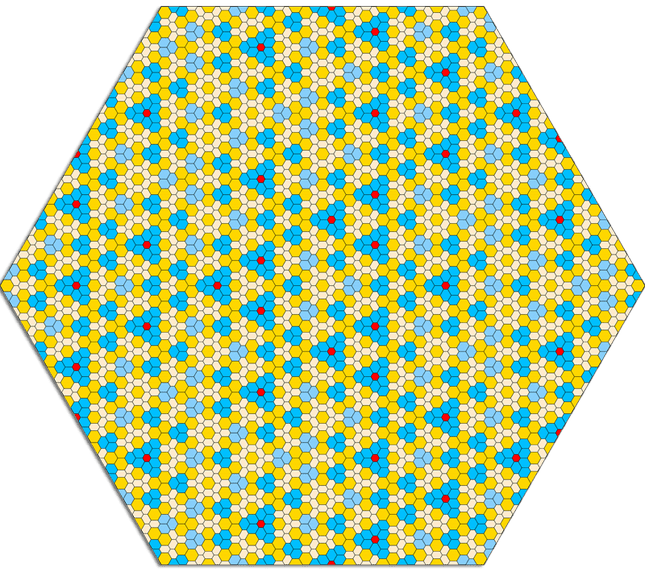That year after college I was kind of shiftless. I chose to just not participate in the whole interview circus that normally accompanies one’s senior year of MIT and let myself graduate without having plans of any kind for the future. I think that I was tired mostly and was kind of sick of the universe of engineering and all of its hurdles and dog and pony shows, and I think I kind of half-understood that this would be the last time in my life in which it would be possible for me to be totally free of that universe — until, I guess, retirement.

Anyway, I lived in the slums of Boston — that is, Roxbury — with a friend of mine from high school and some roommates we found, one of whom ended going to jail (but that is another story), worked temp jobs, and didn’t do much of anything … except for some reason I became obsessed with aperiodic tilings of the plane and substitution tilings generally and put a lot of effort into coming up with one of my own. I wanted to find, for reasons that aren’t clear to me now, an aperiodic analog of the normal regular hexagon tiling.

It’s kind of a blur — I don’t really remember where the above came from as a sequence of steps — but the above is a patch of the best tiling I discovered during this period. It is generated via the following substitutions. The lengths of the sides of triangles and trapezoids are multiples of ϕ, the golden ratio.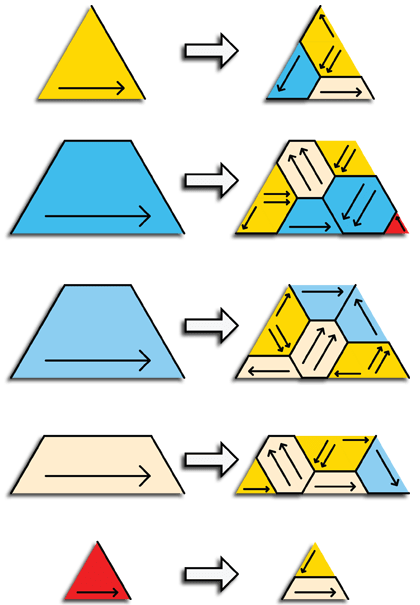As far as I know, the above is original and has not been discussed in the literature but I never was able to come up with local matching rules on the hexagons to enforce aperiodicity.

At that time I did develop software for working on these sorts of structures but what I came up with in retrospect wasn’t The Right Thing. This wasn’t all together my fault. Those were different times: no GitHub, no free code. If I wanted to output into a vector format it would have to be my own vector format. If I wanted to render that format to the screen I would have to write code to render that vector format to the screen, and so on. Also GUI applications were all the rage and were still new and shiny, so I was biased in that direction. I never really liked what I came up with then, and it wasn’t portable anyway; it was a black-and-white Macintosh application in C.

Having the negative example of that project all those years ago made it easy to see what I actually needed: not a GUI application but a programming language. So last week, I wrote one: a little language for specifying recursive structures like the above and rendering them in SVG. I’m calling it Snurtle because it is basically a combination of Python (a snake) and the turtle-based graphics of Logo. I chose Python syntax because I wrote the Snurtle interpreter in Python and thus got tokenization for free using Python’s “tokenize” module.

So, for example, the following simple substitution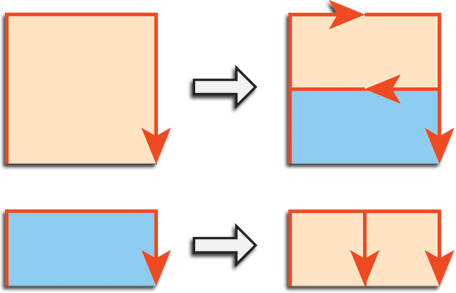is represented by the following Snurtle script:

```sub square(n):
terminal:
poly("bisque", "orangered", 1 ):
forward(n)
turn(PI/2)
forward(n)
turn(PI/2)
forward(n)
nonterminal:
rectangle(n)
branch:
turn(PI)
forward(n/2)
turn(-PI/2)
forward(n/2)
square(n/2)
square(n/2)

sub rectangle(n):
terminal:
poly("lightskyblue", "orangered", 1 ):
forward(n/2)
turn(PI/2)
forward(n)
turn(PI/2)
forward(n/2)
nonterminal:
square(n/2)
turn(PI)
square(n/2)
```

yielding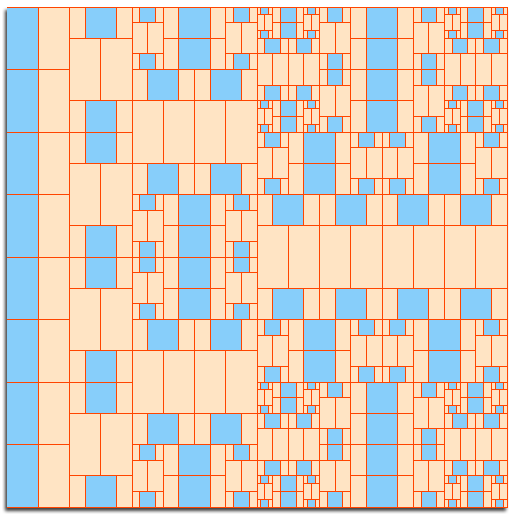which, I think, is self-explanatory except for the “branch” block. Branch blocks tell Snurtle to push the state of the turtle on to a stack and then pop it when exiting the branch i.e. branch works like parentheses operators in L-systems. Also the following Snurtle constructs are not illustrated in the above:

• flip blocks: similar in syntax to branch above. Tell Snurtle to multiply the angle arguments passed to turn statements by -1. (i.e. flipping them)
• stroke and fill blocks: similar to “poly” above.

Anyway, here is my snurtle source code. Usage to generate the above would be:

snurtle.py -w -s square -k 500 -m 8 -o squares.html -c “10,10” “snurtle_scripts\squares.snu

where

• -w : wrap the generated SVG in HTML (so it can be viewed in browser)
• -s : initital substitution used to kick off the recursion
• -k : scale factor in SVG output
• -m : max stack depth before substituting in terminal blocks rather than nonterminal and ending the recursion
• -o : output filename
• c : starting coordinate of the turtle.
• -d : Comma delimited string as -c, that provides width and height attributes for the SVG. (not shown)

Snurtle is pretty rough at this point, but I plan to continue working on it, especially if there is interest. Check back here, The Curiously Recurring Gimlet Pattern, for updates — or on this Quora blog which I will try to keep in sync — if you are interested. In particular, I plan on adding the following features / dealing with the following issues:

• Substitutions can’t currently have more than one argument. I just never got around to adding this functionality as I never had a use-case in all the sample scripts I have tried, but there is no reason to limit “sub” blocks in this way.
• Color, stroke color, and stroke thickness parameters to poly, stroke and fill blocks should be optional with intelligent defaults but aren’t currently.
• Maybe add a z-order parameter to poly, stroke, and fill.
• Add variables.
• Possibly add “mirror” blocks which would be syntactic sugar for executing the block’s body in a flipped branch and then executing it again normally. This would be handy in definitions of complicated structures like my golden hexagon substitution.
• Add “reverse” blocks which would cause the statements in a block to be executed in last to first order, recursively running compound statements this way too.
• Add some kind of loop control structure, “repeat” or something.
• Built-in system variables for (like “PI” above) for current stack depth and max stack depth.
• Add exponentiation to the set of operations that can be performed in expressions.

# The World’s Simplest 2D Curve Library

For the game I’m working on I need to have sprites that travel along curving paths.

I’m talking about the sprites traveling along somewhat arbitrary curves, meaning curves that look good, not curves that result from gravity or other physical forces. If you need those kinds of curves, e.g. the parabolic trajectories of cannonballs, you need to simulate the forces acting on the sprites and that is not what I’m talking about in this post.

Caveat aside, an arbitrary curving path is a pretty common thing to need but I think is unnecessarily headache-inducing because curves in graphics are just confusing. Maybe you’ve found yourself thinking

• I don’t know what the difference between a spline, a bezier curve, a bezier curve of various degrees, a B-spline, a t-spline, etc. is.
• I don’t know which of the things mentioned above I need.
• Every time I try read the wikipedia article on these things the math gets heavy and my eyes glaze over

etc.?

So assuming it’s not just me, as a public service I’m going to try to clear this up.

Short version, if you need to have a sprite that travels along a curving path from point A to point B in x amount of time, you probably need a cubic bezier curve and generally, in 2d game programming, all you will ever need probably is n cubic bezier curves possibly concatenated together. You can concatenate them yourself if you need to do that, so what you need is a way to define a cubic bezier and a function get the point along the bezier at some time t. Despite what you would think from trying read the literature, this turns out to be trivial — I mean less than a dozen lines of code.

More thoroughly, explaining away my bulleted list above:

• A spline is a more general term than a “bezier curve”: a bezier curve is a particular polynomial function (that I will implement below) that defines a curve that goes from point A to point B given some control points. A bezier spline is an aggregation of n of these. A general spline can be an aggregation of other kinds curves e.g. a B-spline is composed of a bunch of curves that are generalizations of bezier curves.
• The only kinds of beziers you need to be concerned with are quadratic and cubic beziers. Quadratic beziers are just parabolas and are not interesting. Cubic beziers are curves that go from point A to point B and are tangent to a given line at A and tangent to given line at B. They are defined by A and B plus two other control points that define the tangent lines and the weight they have on the curve.
• Cubic bezier curves are easy to implement. See below.

So here is my curve “library”:
Bezier.h

```#include &lt;utility&gt;

class Bezier {
private:
float x1_, y1_, x2_, y2_, x3_, y3_, x4_, y4_;
public:
Bezier(float x1, float  y1, float x2, float y2, float x3, float y3, float x4, float y4);
std::pair&lt;float,float&gt; getPoint(float t) const;
};
```

Bezier.cpp

```#include &quot;Bezier.h&quot;

Bezier::Bezier(float x1, float  y1, float x2, float y2, float x3, float y3, float x4, float y4) :
x1_(x1), y1_(y1),
x2_(x2), y2_(y2),
x3_(x3), y3_(y3),
x4_(x4), y4_(y4) {
}

std::pair&lt;float,float&gt; Bezier::getPoint(float t) const {
float x = (x1_+t*(-x1_*3+t*(3*x1_ - x1_*t))) + t*(3*x2_+t*(-6*x2_ + x2_*3*t)) + t*t*(x3_*3-x3_*3*t) + x4_*t*t*t;
float y = (y1_+t*(-y1_*3+t*(3*y1_ - y1_*t))) + t*(3*y2_+t*(-6*y2_ + y2_*3*t)) + t*t*(y3_*3-y3_*3*t) + y4_*t*t*t;
return std::pair&lt;float,float&gt;(x,y);
}
```

You define a cubic bezier by making a Bezier object giving the constructor four points. (x1,y1) and (x4,y4) will be the start and end of the curve. The curve will be tangent to line segment (x1,y1)-(x2,y2) at its start and tangent to (x3,y3)-(x4,y4) at the end. To get a point along the curve call getPoint(t) where t=0.0 gives you (x1,y1), t=1.0 gives you (x4,y4), and 0.0 < t < 1.0 gives you the point along the curve in which 100t percent of the curve has been traversed e.g. 0.5 is halfway.

So that’s it. Code is here. I also included a Win32 GDI project that draws cubic beziers, screenshot below. (The sample program is also a little example of how to write a very basic Win32 program, which these days younger programmers seem to appreciate as a sort of parlor trick…)# Marble Madness Madness

gamedev.net recently linked to this video about the making of Marble Madness, which got me thinking about the raster-to-vector via contour extraction script I wrote in Python last year and the fact that, it being the future and all, I can probably find all of the art from Marble Madness unrolled into a single image file. So three clicks later and, oh yeah: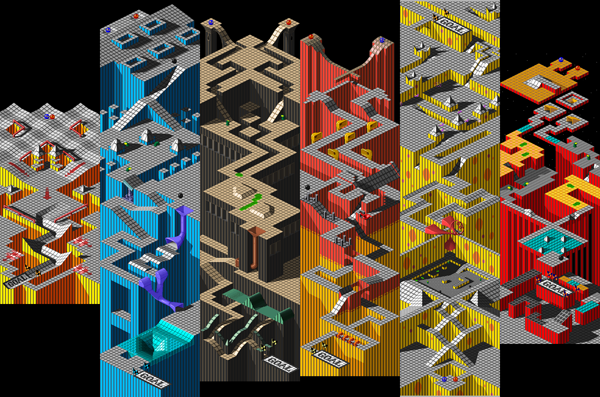(Click the image for full size)

So I ran the above through my raster-to-vector converter. Here are the results (zipped, this is a huge file, over 20,000 SVG paths)  This file kills Adobe Illustrator. It took 15 minutes just to open it.

SVG for a single level is more manageable.  Here’s  the 2nd level as unzipped SVG … (curious to see if various browsers can handle this) Illustrator could handle this one pretty well so I experimented with applying various vector filters. Below is a bit of it with the corners rounded on the paths (click for a larger version):Not sure what this all amounts to … just some stuff I did today. However I did learn

• Python is slow. It took a really long time to generate the big file, seemed too long. I think C++ would’ve been like an order of magnitude faster — that’s my intuition anyway.
• My contour extraction program really works which is kind of surprising — I thought for sure running it on something like this would crash it. (It does still have the problem that it can’t handle paletted raster image formats, but that’s the only bug I encountered)